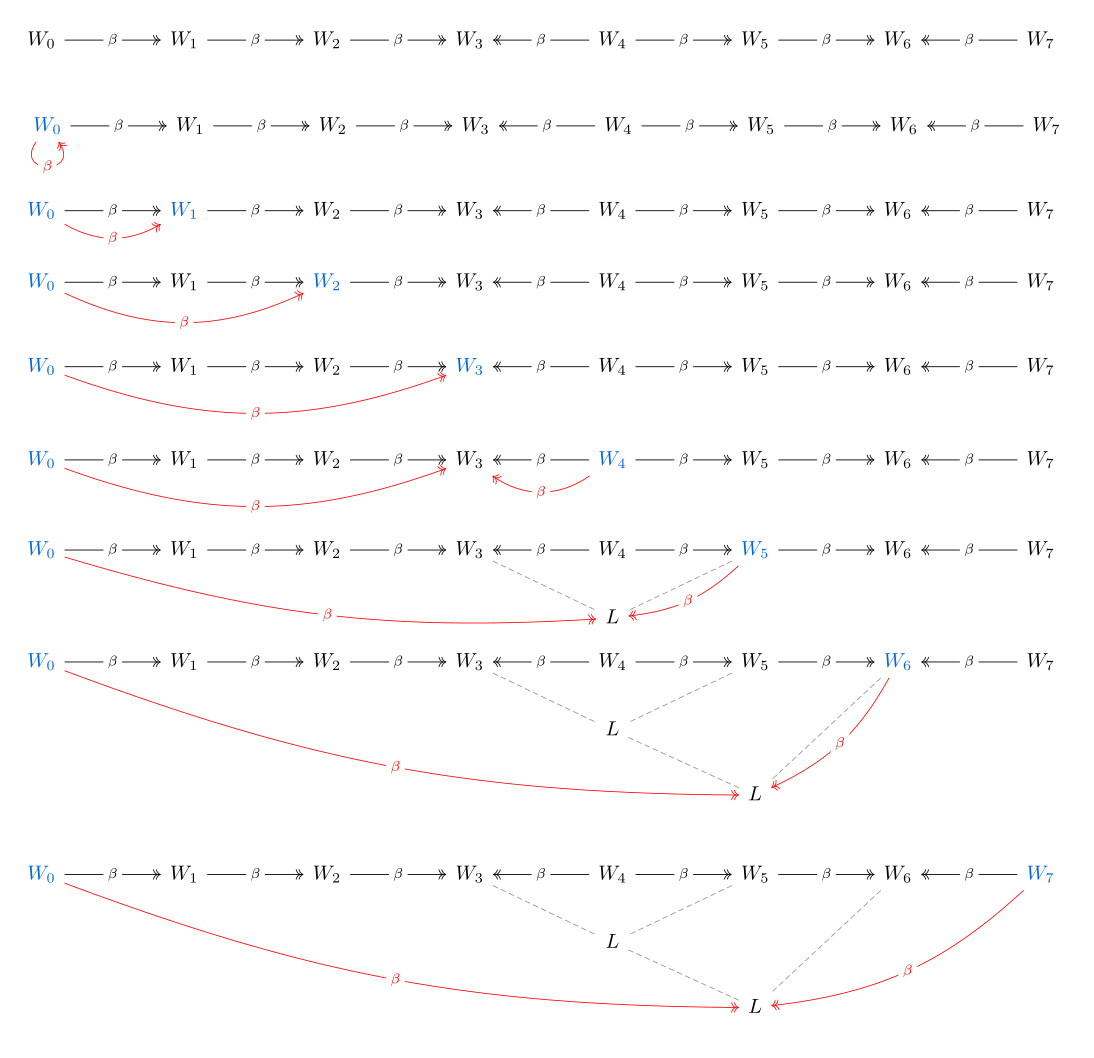Show Question
Math and science::Theory of Computation::Lambda calculus

# Confluence

### Confluence

Let $$M$$ be a lambda term. If $$M \multiBetaReduction N_1$$ and $$M \multiBetaReduction N_2$$ for some lambda terms $$N_1$$ and $$N_2$$, then there exists a lambda term $$N_3$$ such that both $$N_1 \multiBetaReduction N_3$$ and $$N_2 \multiBetaReduction N_3$$.

Confluence is also called the Church-Rosser theorem.

There are three corollaries of the confluence theorem listed on the back side. Can you remember them?

Confluence is a defining theorem in untyped lambda calculus. It shows that the result of a computation is independent of the path taken during computation—paths differ depending on the choice of redex to reduce first.

The proof of confluence is unexpectedly complicated, and hints at lambda calculus not being the best theory in which to express the theorem.

### Corollaries

The following three results are typically proved using confluence. Their simplicity might deceive readers into thinking they can be proved easily without using confluence.

#### Corollary 1

Let $$M$$ and $$U$$ be lambda terms.

Suppose that $$M \betaEq U$$. Then there exists a lambda term $$L$$ such that $$M \multiBetaReduction L$$ and $$U \multiBetaReduction L$$.

Some intuition. Imagine a bi-directional reduction sequence. Nodes like $$W \leftarrow X \rightarrow Y$$ can be replaced with $$W \rightarrow X' \leftarrow Y$$ using confluence. Repeating this reduction will eventually create a sequence like:

$M \rightarrow W_1 \rightarrow ... W_k \leftarrow W_{k+1} \leftarrow W_{k+2} ... \leftarrow U$

The actual proof uses recursion.

Proof. Let $$M$$ and $$U$$ be lambda terms and assume that $$M \betaEq U$$. By this assumption, there exists a bi-directional reduction sequence, $$(W_n)_{n=0}^{N}$$ from $$M$$ to $$U$$. Induce on the length of this sequence, $$N$$.

Base case, $$N = 0$$. If $$N = 0$$, then $$M \equiv U$$. So it is true that $$M \multiBetaReduction M$$ and $$U \multiBetaReduction M$$, so $$M$$ meets the criteria of the lambda term we are seeking (so does $$U$$).

Assume that the statement is true for some sequence length $$k > 0$$. Then consider $$k + 1$$. First note that $$W_{k+1} \equiv U$$, by the definition of a bi-direction reduction sequence from $$M$$ to $$U$$. The sub-sequence $$(W_n)_{n=0}^{k}$$ connects $$M$$ and $$W_k$$, meaning $$M \betaEq W_k$$, and so, by the induction assumption, there exists a lambda term $$L$$ such that $$M \multiBetaReduction L$$ and $$W_k \multiBetaReduction L$$. From here we consider two cases: it is the case that either $$W_k \betaReduction U$$ or $$U \betaReduction W_k$$.

• If $$W_k \betaReduction U$$ we can use confluence to assert that there exists another lambda term $$L'$$ such that both $$L \multiBetaReduction L'$$ and $$U \multiBetaReduction L'$$. By transitivity of multi-step beta reduction, $$M \multiBetaReduction L'$$. Thus, $$L'$$ is the lambda term that fits the induction requirement.
• If $$U \betaReduction W_{k}$$, then $$U \multiBetaReduction L$$, by associativity of the multi-step beta reduction, and $$L$$ is the term that fits the induction requirement.

#### Corollary 2

Let $$M$$ and $$U$$ be lambda terms.

If $$M$$ has $$U$$ as a β-normal form, then $$M \multiBetaReduction U$$.

Proof. Let $$M$$ and $$U$$ be lambda terms and assume that $$M$$ has $$U$$ as a β-normal form. By the previous lemma, there is a lambda term $$L$$ such that $$M \multiBetaReduction L$$ and $$U \multiBetaReduction L$$. Being in normal form, $$U \multiBetaReduction L$$ can only be true if $$U \equiv L$$.

#### Corollary 3

A lambda term has at most one β-normal form.

Proof. Proof by contradiction. Assume $$M$$ is a lambda term with two normal forms, $$U_1$$ and $$U_2$$. By confluence, there exists a $$U_3$$ such that $$U_1 \multiBetaReduction U_3$$ and $$U_2 \multiBetaReduction U_3$$. Being normal forms, both $$U_1$$ and $$U_2$$ must be equivalent to $$U_3$$, and thus, they must be equivalent to each other. This contradicts the assumption that there were two different normal forms.

### Corollary 1 visualization

The following figure tries to illustrate how an algorithm can move along a bi-directional reduction sequence and calculate the term that can be reached by both ends in a single direction. Confluence is invoked where the grey dashed lines are seen.